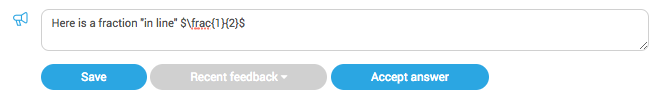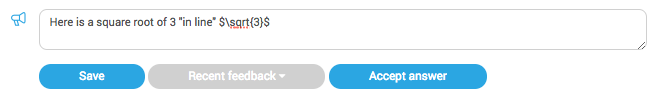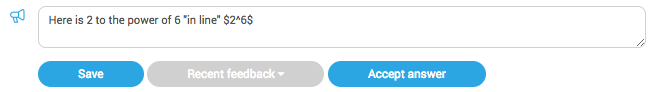You may be familiar with LaTeX from college- it's a maths markup language that is commonly used on computers. We use it in HegartyMaths when displaying questions and we also use it for you to give mathematics in your feedback to students.

A few written instructions are given below, but you can try it out for yourself using our LaTeX helper.

What LaTex does to your feedback:

In order for your student to see feedback like this:In the feedback field, you write this:How it works:

If you want to put fractions, powers, square roots and so on into your feedback, this is how to do it:

Very simply, use dollar signs ($) at the start and end of your code.$x$will look likeMultiplying and dividing: \div and \times are used to enter divide and multiply symbols: Teacher writes:Student sees:Teacher writes:Student sees:Fractions To do fractions, use the keyword "\frac" A half is$\frac{1}{2}$Teacher writes:Student sees:Square roots To do square roots, use the keyword \sqrt The square root of 3 is$\sqrt{3}$Teacher writes:Student sees:Powers and indices Use ^ to give powers 2 to the power of 6 is$2^6\$

Teacher writes:Student sees:For negative indices put the index in curly brackets {} like so:

Teacher writes:Student sees:Finally

If you want the maths to stand on its own line, use double dollars at the start and end of the phrase.

Teacher writes:Student sees: